# How to calculate time in Excel - time difference, adding / subtracting times

This tutorial focuses on various ways to calculate times in Excel. You will find a few useful formulas to add and subtract times, calculate time difference, or elapsed time, and more.

In the last week's article, we had a close look at the specificities of Excel time format and capabilities of basic time functions. Today, we are going to dive deeper into Excel time calculations and you will learn a few more formulas to efficiently manipulate times in your worksheets.

## How to calculate time difference in Excel (elapsed time)

To begin with, let's see how you can quickly calculate elapsed time in Excel, i.e. find the difference between a beginning time and an ending time. And as is often the case, there is more than one formula to perform time calculations. Which one to choose depends on your dataset and exactly what result you are trying to achieve. So, let's run through all methods, one at a time.

#### Formula 1. Subtract one time from the other

As you probably know, times in Excel are usual decimal numbers formatted to look like times. And because they are numbers, you can add and subtract times just as any other numerical values.

The simplest and most obvious Excel formula to calculate time difference is this:

=End time - Start time

Depending on you data structure, the actual time difference formula may take various shapes, for example:

 Formula Explanation `=A2-B2` Calculates the difference between the time values in cells A2 and B2. `=TIMEVALUE("8:30 PM") - TIMEVALUE("6:40 AM")` Calculates the difference between the specified times. `=TIME(HOUR(A2), MINUTE(A2), SECOND(A2)) - TIME(HOUR(B2), MINUTE(B2), SECOND(B2))` Calculates the time difference between values in cells A2 and B2 ignoring the date difference, when the cells contain both the date and time values.

Remembering that in the internal Excel system, times are represented by fractional parts of decimal numbers, you are likely to get the results similar to this: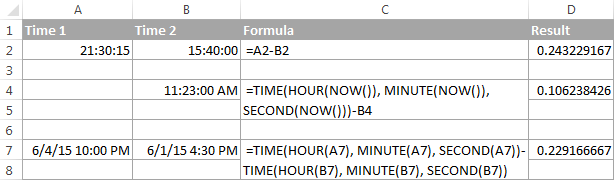The decimals in column D are perfectly true but not very meaningful. To make them more informative, you can apply custom time formatting with one of the following codes:

 Time code Explanation h Elapsed hours, display as 4. h:mm Elapsed hours and minutes, display as 4:10. h:mm:ss Elapsed hours, minutes and seconds, display as 4:10:20.

To apply the custom time format, click Ctrl + 1 to open the Format Cells dialog, select Custom from the Category list and type the time codes in the Type box. Please see Creating a custom time format in Excel for the detailed steps.

And now, let's see how our time difference formula and time codes work in real worksheets. With Start times residing in column A and End times in column B, you can copy the following formula in columns C though E:

`=\$B2-\$A2`

The elapsed time is displayed differently depending on the time format applied to the column: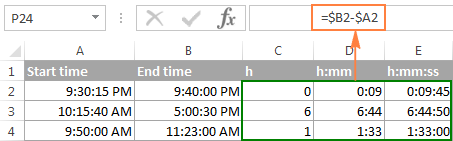Note. If the elapsed time is displayed as hash marks (#####), then either a cell with the formula is not wide enough to fit the time or the result of your time calculations is a negative value.

#### Formula 2. Calculating time difference with the TEXT function

Another simple technique to calculate the duration between two times in Excel is using the TEXT function:

• Calculate hours between two times:

`=TEXT(B2-A2, "h")`

• Return hours and minutes between 2 times:

`=TEXT(B2-A2, "h:mm")`

• Return hours, minutes and seconds between 2 times:

`=TEXT(B2-A2, "h:mm:ss")`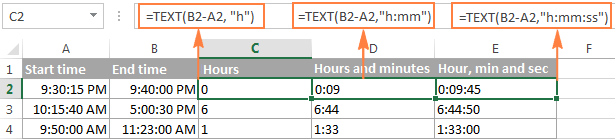Notes:

• The value returned by the TEXT function is always text. Please notice the left alignment of text values in columns C:E in the screenshot above. In certain scenarios, this might be a significant limitation because you won't be able to use the returned "text times" in other calculations.
• If the result is a negative number, the TEXT formula returns the #VALUE! error.

#### Formula 3. Count hours, minutes or seconds between two times

To get the time difference in a single time unit (hours ,minutes or seconds), you can perform the following calculations.

Calculate hours between two times:

To present the difference between two times as a decimal number, use this formula:

=(End time - Start time) * 24

Supposing that your start time is in A2 and end time in B2, you can use a simple equation B2-A2 to calculate the difference between two times, and then multiply it by 24, which is the number of hours in one day:

`=(B2-A2) * 24`

To get the number of complete hours, use the INT function to round the result down to the nearest integer:

`=INT((B2-A2) * 24)`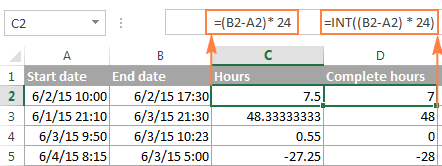Total minutes between two times:

To calculate the minutes between two times, multiply the time difference by 1440, which is the number of minutes in one day (24 hours * 60 minutes = 1440).

=(End time - Start time) * 1440

As demonstrated in the following screenshot, the formula can return both positive and negative values, the latter occur when the end time is less than the start time, like in row 5:

`=(B2-A2)*1440`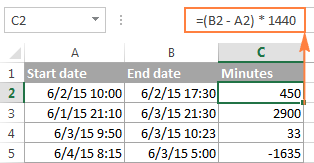Total seconds between times:

To get the total seconds between two times, you multiply the time difference by 86400, which is the number of seconds in one day (24 hours * 60 minutes * 60 seconds = 86400).

=(End time - Start time) * 86400

In our example, the formula is as follows:

`=(B2-A2)* 86400`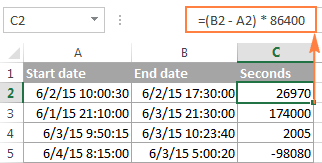Note. For the results to display correctly, the General format should be applied to the cells with your time difference formula.

#### Formula 4. Calculate difference in one time unit ignoring others

To find the difference between 2 times in a certain time unit, ignoring the others, use one of the following functions.

• Difference in hours, ignoring minutes and seconds:

`=HOUR(B2-A2)`

• Difference in minutes, ignoring hours and seconds:

`=MINUTE(B2-A2)`

• Difference in seconds, ignoring hours and minutes:

`=SECOND(B2-A2)`

When using Excel's HOUR, MINUTE and SECOND functions, please remember that the result cannot exceed 24 for hours and 60 for minutes and seconds.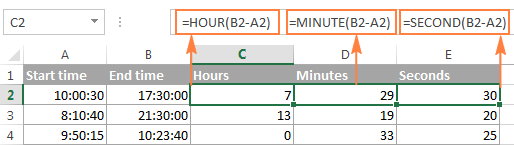Note. If the end time is less than the start time (i.e. the result of the formula is a negative number), the #NUM! error is returned.

#### Formula 5. Calculate elapsed time from a start time to now

In order to calculate how much time has elapsed since the start time to now, you simply use the NOW function to return today's date and the current time, and then subtract the start date and time from it.

Supposing that the beginning date and time is in call A2, the formula below returns the following results, provided you've applied an appropriate time format to column B, h:mm in this example:

`=NOW()-A2`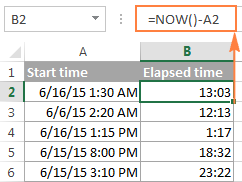In case the elapsed time exceeds 24 hours, use one of these time formats, for example d "days" h:mm:ss like in the following screenshot: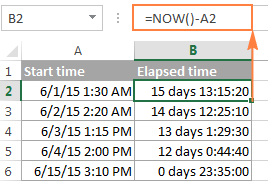If your starting points contain only time values without dates, you need to use the TIME function to calculate the elapsed time correctly. For example, the following formula returns the time elapsed since the time value in cell A2 up to now:

`=TIME(HOUR(NOW()), MINUTE(NOW()), SECOND(NOW())) - A2`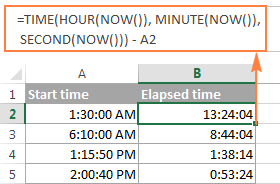Note. The elapsed time is not updated in real-time, it refreshes only when the workbook is reopened or recalculated. To force the formula to update, press either Shift + F9 to recalculate the active spreadsheet or hit F9 to recalculate all open workbooks.

#### Formula 5. Display time difference as "XX days, XX hours, XX minutes and XX seconds"

This is probably the most user-friendly formula to calculate time difference in Excel. You use the HOUR, MINUTE and SECOND functions to return corresponding time units and the INT function to compute the difference in days. And then, you concatenate all these functions in a single formula along with the text labels:

`=INT(B2-A2) & " days, " & HOUR(B2-A2) & " hours, " & MINUTE(B2-A2) & " minutes and " & SECOND(B2-A2) & " seconds"`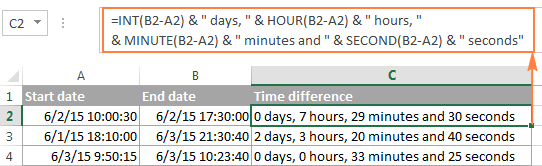To instruct your Excel time difference formula to hide zero values, embed four IF functions into it:

`=IF(INT(B2-A2)>0, INT(B2-A2) & " days, ","") & IF(HOUR(B2-A2)>0, HOUR(B2-A2) & " hours, ","") & IF(MINUTE(B2-A2)>0, MINUTE(B2-A2) & " minutes and ","") & IF(SECOND(B2-A2)>0, SECOND(B2-A2) & " seconds","")`

The syntax may seem excessively complicated, but it works :)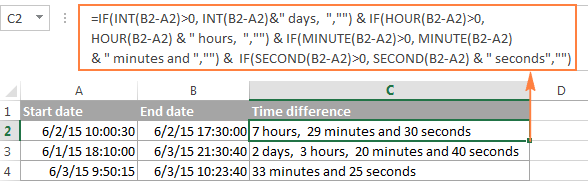Alternatively, you can calculate time difference by simply subtracting the start time from the end time (e.g. `=B2-A2`), and then apply the following time format to the cell:

d "days," h "hours," m "minutes and" s "seconds"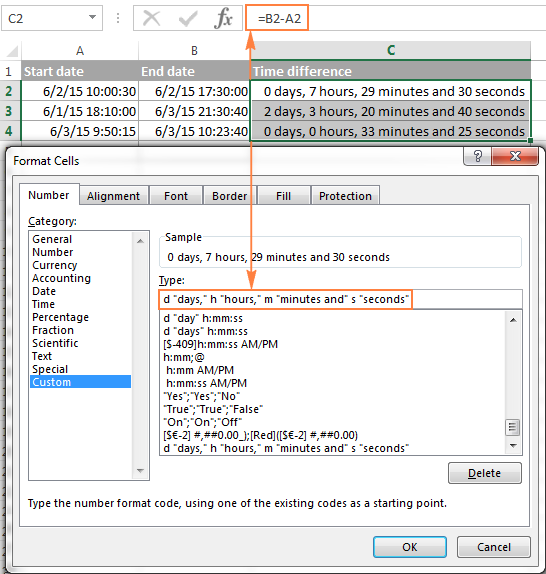An advantage of this approach is that your result would be a normal time value that you could use in other time calculations, while the result of the complex formula discussed above is a text value. A drawback is that the custom time format cannot distinguish between zero and non-zero values and ignore the latter. To display the result in other formats, please see How to show time over 24 hours, 60 minutes, 60 seconds.

## How to calculate and display negative times in Excel

When calculating the time difference in Excel, you may sometimes get the result as ###### error because the difference is a negative time. But is there a way to show negative times properly in Excel? Of course, there is a way, and even more than one :)

#### Method 1. Change Excel Date System to 1904 date system

The fastest and easiest way to display negative time normally (with a minus sign) is switching to the 1904 date system. To do this, click File > Options > Advanced, scroll down to the When calculating this workbook section and put a tick in the Use 1904 date system box.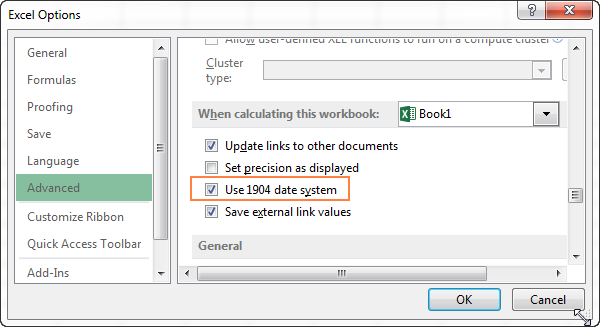Click OK to save the new settings, and from now on negative times will be displayed correctly, like negative numbers: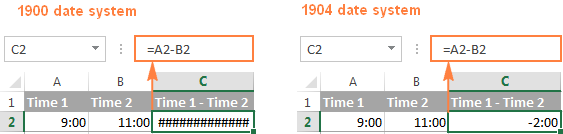#### Method 2. Calculate negative time in Excel with formulas

Is changing Excel's default Date System is not an option, then you can force negative times to display properly using one of the following formulas:

`=IF(A2-B2>0, A2-B2, "-" & TEXT(ABS(A2-B2),"h:mm"))`

`=IF(A2-B2>0, A2-B2, TEXT(ABS(A2-B2),"-h:mm"))`

Both formulas check if the time difference (A2-B2) is greater than 0, and if it is they return that difference. If the time difference is less than zero, the first formula calculates the absolute difference and concatenates the minus sign. The second formula yields exactly the same result by using a negative time format "-h::mm".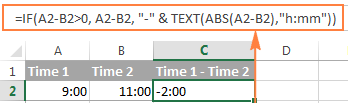Note. Please keep in mind that unlike the first method that treats negative times as negative numeric values, the result of the TEXT function is always a text string that cannot be used in calculations or other formulas.

## Adding and subtracting time in Excel

Basically, there are 2 ways to add and subtract time in Excel:

• Using the TIME function
• Using arithmetic calculations based on the number of hours (24), minutes (1440) and seconds (86400) in one day

The `TIME(hour, minute, second)` function makes Excel time calculations really easy, however it does not allow adding or subtracting more than 23 hours, or 59 minutes, or 59 seconds. If you are working with bigger time intervals, then use one of the arithmetic calculations demonstrated below.

### How to add or subtract hours to time in Excel

To add hours to a given time in Excel, you can use one the following formulas.

TIME function to add under 24 hours

=Start time + TIME(N hours, 0, 0)

For example, if your start time is in cell A2, and you want to add 2 hours to it, the formula is as follows:

`=A2 + TIME(2, 0, 0)`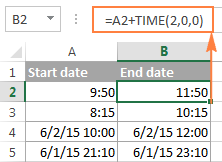Note. If you try adding more than 23 hours with the TIME function, the specified hours will be divided by 24 and the remainder will be added to the start time value. For example, if you try to add 25 hours to "6/2/2015 10:00 AM" (cell A4) using the formula `=A4 + TIME(25, 0, 0)`, the result will be "06/02/2015 11:00", i.e. A4 + 1 hour.

Formula to add any number of hours (under or over 24 hours)

The following formula has no limitations to the number of hours you want to add:

= Start time + (N hours / 24)

For example, to add 28 hours to the start time in cell A2, enter the following formula:

`=A2 + (28/24)`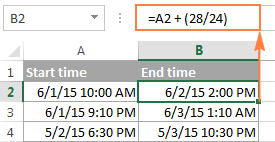To subtract hours from a given time, you use analogous formulas, and just replace "+" with the minus sign:

For example, to subtract 3 hours from the time in cell A2, either of the following formulas will do:

`=A2-(3/24)`

`=A2-TIME(3,0,0)`

To subtract more than 23 hours, use the first one.

### How to add / subtract minutes to time in Excel

To add minutes to a given time, employ the same techniques that we've just used for adding hours.

To add or subtract under 60 minutes

Use the TIME function and supply the minutes you want to add or subtract in the second argument:

=Start time + TIME(0, N minutes, 0)

And here are a couple of real-life formulas to calculate minutes in Excel:

To add 20 minutes to the time in A2: =A2 + TIME(0,20,0)

To subtract 30 minutes from the time in A2: =A2 - TIME(0,30,0)

To add or subtract over 60 minutes

In your calculation, divide the number of minutes by 1440, which is the number of minutes in a day, and add the quotient to the start time:

=Start time + (N minutes / 1440)

To subtract minutes from time, simply replace plus with the minus sign. For example:

To add 200 minutes: `=A2 + (200/1440)`

To subtract 300 minutes: `=A2 -(300/1440)`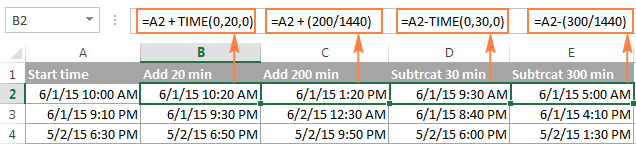### How to add / subtract seconds to a given time

Second calculations in Excel are done in a similar fashion.

To add under 60 seconds to a given time, you can use the TIME function:

=Start time + TIME(0, 0, N seconds)

To add more than 59 seconds, use the following formula:

=Start time + (N seconds / 86400)

To subtract seconds, utilize the same formulas with the minus sign (-) instead of plus (+).

In your Excel worksheets, the formulas may look similar to these:

To add 30 seconds to A2: `=A2 + TIME(0,0,31)`

To add 1200 seconds to A2: `=A2 + (1200/86400)`

To subtract 40 seconds from A2: `=A2 - TIME(0,0,40)`

To subtract 900 seconds from A2: `=A2 - (900/86400)`

## How to sum time in Excel

The Excel sum time formula is the usual SUM function, and applying the proper time format to the result is what does the trick.

Supposing you have a few project times in column B and you want to add them up. You write a simple SUM formula like the one below and get the result in the default format such as hh:mm:ss.

`=SUM(B2:B4)`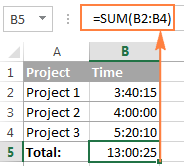In some cases the default time format works just fine, but sometimes you may want more, for example to display the total time as minutes and seconds, or seconds only. The good news is that no other calculations are required, all you have to do is apply custom time format to the cell with the SUM formula.

Right click the cell and select Format Cells in the context menu, or press Ctrl + 1 to open the Format Cells dialog box. Select Custom from the Category list and type one of the following time formats in the Type box:

• To display total time as minutes and seconds: [m]:ss
• To display total time as seconds: [ss]

The result will look as follows: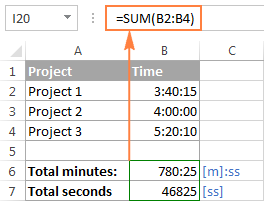### How to sum over 24 hours in Excel

In order to add up more than 24 hours, you use the same SUM formula as discussed above, and apply one of the following time formats to the cell:

 Format Displays as Explanation [h]:mm 30:10 Hours and minutes [h]:mm:ss 30:10:20 Hours, minutes and seconds [h] "hours", mm "minutes", ss "seconds" 30 hours, 10 minutes, 20 seconds d h:mm:ss 1 06:10:20 Days, hours, minutes and seconds d "day" h:mm:ss 1 day 06:10:20 d "day," h "hours," m "minutes and" s "seconds" 1 day, 6 hours, 10 minutes and 20 seconds

To see how these custom time formats may look like in your Excel worksheet, please have a look at the screenshot below, where the same SUM formula is entered in cells A9 to A13:

`=SUM(\$B\$2:\$B\$4)`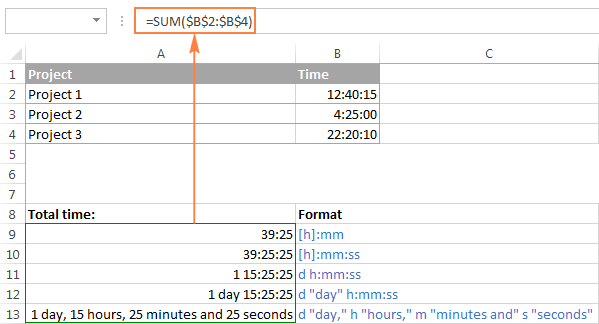Note. The above custom time formats work for positive values only. If the result of your time calculations is a negative number, e.g. when you are subtracting a bigger time from a smaller time, the result will be displayed as #####. To display negative times differently, please see custom format for negative time values.

Also, please keep in mind that the time format applied to a cell changes only the display presentation without changing the cell's value. For example, in the screenshot above, cell A13 looks like text, but in fact it's a usual time value, which is stored as a decimal in the internal Excel system. Meaning, you are free to refer to that cell in other formulas and calculations.

For more information, please see How to calculate and show over 24 hours, 60 minutes, 60 seconds.

## Date & Time Formula Wizard - quick way to calculate times in Excel

Now that you know a bunch of different formulas to add and subtract times in Excel, let me show you the tool that can do it all. Okay, almost all :)

Here comes Ablebit's Date & Time Formula Wizard for Excel:In the Date & Time Wizard dialog window, you switch to the Add or Subtract tab, depending on which operation you want to perform, and do the following:

• Click the Show time fields link in the left part of the window.
• Supply values or cell references for the formula arguments. As you fill in the argument boxes, the wizard builds the formula in the selected cell.
• When finished, click the Insert Formula

That's it! For example, this is how you can add the specified number of hours, minutes and seconds to the time in A4: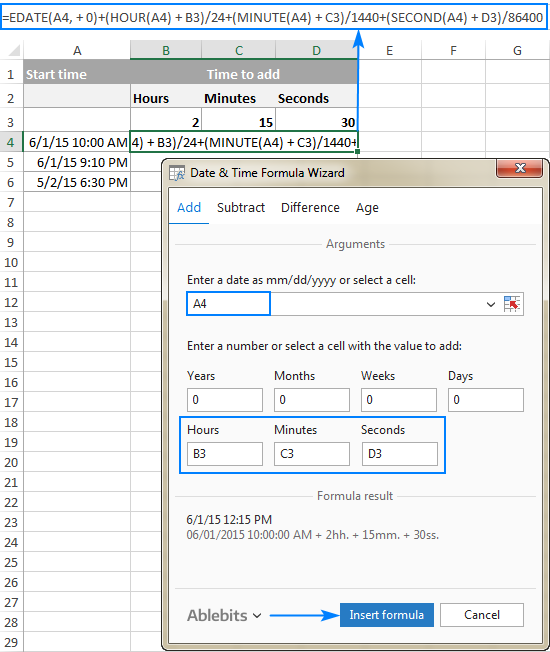If you need to copy the formula to other cells, fix all references except the cell containing the original time (A4) with the \$ sign like shown in the screenshot below (by default, the wizard always uses relative references). Then double-click the fill handle to copy the formula down the column and you are good to go!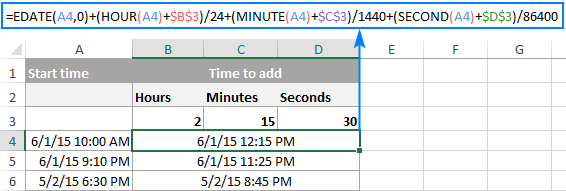Besides time calculations, the wizard can also add and subtract dates, get the difference between two dates, and calculate age from the birthdate.

If you are curious to try this tool in your own worksheets, you are welcome to download the evaluation version of our Ultimate Suite below.

This is how you calculate time in Excel worksheets. To learn other ways to manipulate dates and times in Excel, I encourage you to check out the resources at the end of this article. I thank you for reading and hope to see you on our blog next week!

Excel Time Calculations - formula examples (.xlsx file)
Ultimate Suite - trial version (.exe file)

### Excel Date and Time tutorials:

1. If a car travel distance 190km @ the speed of 120km/h. it took 1 hour and 35 mint to cover 190km distance. i want result out put as "1 hour 35 min" on excel please help me

2. In time (Column BF14)15/02/2022 2:27 PM and out time (Column BF15)16/02/2022 2:52 PM, how to find the no of hours, minutes between these two dated

3. Hi
I am Raju, looking for solution to get total spent market visit time by an employee. for example, below are the rows for daily spent time, and 3rd column is the daily spent duration, bu how can I get the total cumulative of a week or month auto sum in 3rd columns down. the formula I used between time in and time out is as =TEXT([@[TIME OUT]]-[@[TIME IN]],"h:mm") and need to get total time spend from 3rd column
TIME IN TIME OUT Total Time Spent
09:00:00 09:30:00 0:30
09:35:00 10:00:00 0:25
10:10:00 10:30:00 0:20

4. how do you add/subtract time with milliseconds? I don't see a formula for this.

IE: m/d/yyyy h.mm.ss.000

5. i'm trying to replicate the example you have in Formula1 but i only get #VALUE!
i am not sure why this happens and i am using the exact same values as you.

6. I'm trying to subtract 500:00 from 15000:00 and it gives me #VALUE!.

7. How do I calculate hours above 10000:00 ?

8. Is there a way to calculate the difference in time between these two values?

Dec 30 2017 4:09PM and Jan 3 2018 9:18AM

Or is there a way to reformat these cells so I can? The date and time are in one single cell.

Thank you,
Jenna

9. Thank u Mr. rajesh peshiya for the reply.
But i need to below format
4:30 - 4:00 = use formula =text(a1-b1,"h:mm") result 0:30 ITS OK
4:40 - 4:40 = use formula =text(a2-b2,"h:mm") result #VALUE! NEED TO RESULT 0:00
4:55 - 5:45 = use formula =text(a3-b3,"h:mm") result #VALUE! NEED TO RESULT -0:50
4:55 - 5:30 = use formula =text(a4-b4,"h:mm") result #VALUE! NEED TO RESULT -0:35
4:30 - 5:00 = use formula =text(a5-b5,"h:mm") result #VALUE! NEED TO RESULT -0:30

Pls Help me..

Regards
Bandara

10. How to calculate
A B C
1 5:20AM-2.20AM= =TEXT(A1-B1,"H:MM") RESULTS 3:00

BUT

2 2:20AM-5:20AM= =TEXT(A1-B1,"H:MM") RESULTS #VALUE! (-3:00)

5 - 2 = 3
3 - 5 = -2 Why didn't work (-) Time

pls Help me

11. Dear Friends i am in problem solving the issue.
i want to make attendance record. requirement is
Fixed Time = 8:30 Am
if employ come to office up to 8:40 its ok, after 8:45 to 10:00 am he will be consider Late, after 10:00 he will be consider Half leave, and if he is absent then formula show the person will be absent.

• Hello,
For me to understand the problem better, please send me a small sample workbook with your source data and the result you expect to get to support@ablebits.com. Please don't worry if you have confidential information there, we never disclose the data we get from our customers and delete it as soon as the problem is resolved.

12. hi,i have problem on time calculation:
have to find the elapsed time example.
start time= 23:45, end time= 02:15.answer must 2:30 .
but it is not work. pls help

• Hello,

If I understand your task correctly, try to use one of the following formulas:

=TIME((HOUR(B1)+24)-HOUR(A1),MINUTE(B1)-MINUTE(A1),0)

or

=(B1+24)-A1

Where cell A1 is “23:45”, cell B1 is “02:15”

13. Thanks a lot man....
=(clr_time-occur_time)*1440 worked for me!

14. hello got an easy one for you.. hopefully.

i have worked out my formula to calculate hours worked in total for a day eg;
06:00 - 16:00 = 10 works fine. however when the time goes past midnight it doesnt work because its a negative eg;
18:00-06:00 should be 12 hours but comes up #########

• Hello,

=(VALUE(A1)+1)-VALUE(B1)

15. Hey,,

Thanks for the information you shared really useful!
But let's say that we have this table
Time of sample Seconds between samples*
9:30 5550
12:35 9450
14:45 6150
16:00 7650
19:00 5400

*assuming start sampling time 9:30 and end sampling time 19:00
How I will find the Seconds between samples as above using formulas in excel??

• Hello,

=TEXT((HOUR(VALUE(A3)-VALUE(A2))*3600)+(MINUTE(VALUE(A3)-VALUE(A2))*60),"@")

16. ABISHA J Nov 22 2017 03:28 Nov 22 2017 03:36 UG STUDENT
ABISHA J Nov 17 2017 04:03 Nov 17 2017 04:40 UG STUDENT
ABISHA J Nov 14 2017 02:56 Nov 14 2017 04:30 UG STUDENT
ABISHA J Nov 13 2017 03:12 Nov 13 2017 04:02 UG STUDENT
ABISHA J Nov 11 2017 11:14 Nov 11 2017 03:27 UG STUDENT
ABISHA J Nov 09 2017 03:13 Nov 09 2017 04:48 UG STUDENT
ABISHA J Nov 08 2017 03:46 Nov 08 2017 05:14 UG STUDENT
ABISHA J Nov 07 2017 03:39 Nov 07 2017 05:47 UG STUDENT
ABISHA J Nov 06 2017 03:42 Nov 06 2017 05:07 UG STUDENT
ABISHA J Nov 02 2017 04:16 Nov 02 2017 05:17 UG STUDENT
how to Calculate total hours
ADARSH VIGNU M Nov 22 2017 03:32 Nov 22 2017 04:02 UG STUDENT
ADARSH VIGNU M Nov 09 2017 02:54 Nov 09 2017 03:24 UG STUDENT
ADARSH VIGNU M Nov 07 2017 03:49 Nov 07 2017 04:37 UG STUDENT
ADARSH VIGNU M Nov 06 2017 03:49 Nov 06 2017 04:47 UG STUDENT
ADARSH VIGNU M Nov 02 2017 04:41 Nov 02 2017 05:21 UG STUDENT
ADARSH VIGNU M Nov 02 2017 03:16 Nov 02 2017 04:28 UG STUDENT

• Hello,

For me to understand the problem better, please send me a small sample workbook with your source data and the result you expect to get to support@ablebits.com. Please don't worry if you have confidential information there, we never disclose the data we get from our customers and delete it as soon as the problem is resolved.

17. Hi,

I have a problem of summing spent time like below
An employee has spent time as below in two ocation

7hr and 48mnt
52 mnts

Altogether should be 8hrs and 40mnts
I have summed as below but it doesnt come

7.48+.52=8

How do i take 8.4 (8hrs and 40 mnts) as answer.

Thank You

• Hello, Ruwanara,

=TIME(7,48,0)+TIME(0,52,0)

18. Hi I am working on a project where I would like to know the time duration between to given dates/times - HOWEVER I would like this to be based off of a work day (8 hours). So for example -

A1:3/13/2017 8:47 AM *Creation Date
B1:3/16/2017 7:29 AM *Closing Date
C1:7:00 AM *Time in each day
D1:4:00 PM *Time out each day

I would also like this to exclude weekends if possible.

I need the time difference between A1 and B1 based off of work day.

Any tips would be appreciated

• Hello, BRITTANY,

=IF(NETWORKDAYS(A1+1,B1-1)>0,NETWORKDAYS(A1+1,B1-1)*8,0)+HOUR(IF(OR(WEEKDAY(A1)=1,WEEKDAY(A1)=7),0,IF((TIME(HOUR(A1), MINUTE(A1),SECOND(A1))-C1)>=(D1-C1),(D1-C1),(TIME(HOUR(A1), MINUTE(A1),SECOND(A1))-C1))))+HOUR(IF(OR(WEEKDAY(B1)=1,WEEKDAY(B1)=7),0,IF((TIME(HOUR(B1), MINUTE(B1),SECOND(B1))-C1)>=(D1-C1),(D1-C1),(TIME(HOUR(B1), MINUTE(B1),SECOND(B1))-C1)))) & " hours " & MINUTE(IF(OR(WEEKDAY(A1)=1,WEEKDAY(A1)=7),0,IF((TIME(HOUR(A1), MINUTE(A1),SECOND(A1))-C1)>=(D1-C1),(D1-C1),(TIME(HOUR(A1), MINUTE(A1),SECOND(A1))-C1)))) + MINUTE(IF(OR(WEEKDAY(B1)=1,WEEKDAY(B1)=7),0,IF((TIME(HOUR(B1), MINUTE(B1),SECOND(B1))-C1)>=(D1-C1),(D1-C1),(TIME(HOUR(B1), MINUTE(B1),SECOND(B1))-C1)))) & " minutes"

19. WHAT FORMULA DID I USE FOR THIS FORMAT

16:00 22:00 = 5

• Hello, MARCO,
=HOUR(TIME(22,0,0)-TIME(16,0,0))

20. I have tried different formulas to get diference in days/hrs but due to following date/time format Im getting stuck can you please help 9each date & time is in the same 1 x cell
2017.10.03 04:03:27 2017.10.04 16:49:50

Regards

21. Hi

I'm trying to produce a production schedule for a manufacturing plant how can I add production-time to calculate finish time/date. From this I need to subtract weekends and non production hours ie 10pm-6am.

Example

Start date & Time = 8/10/17 & 06:10
Total production time = 148hrs
End date & time (minus weekends and hours between 10pm-6am) = ?

• Hi, Dave,

our tech specialist has come up with the following formulas for you:
1) if A1 contains start date & time, and A2 contains 148hrs, here's a formula to show day/month/year:
=TEXT(IF(VALUE(TIME(HOUR(\$A\$1),MINUTE(\$A\$1),SECOND(\$A\$1)))+VALUE(TIME(HOUR(\$A\$2/(22-6)),0,0))<=VALUE(TIME(22,0,0)),WORKDAY.INTL(\$A\$1,INT(\$A\$2/(22-6)))+((\$A\$2/(22-6))-INT(\$A\$2/(22-6)))+VALUE(TIME(IF(HOUR(\$A\$1)<6,6,HOUR(\$A\$1)),MINUTE(\$A\$1),SECOND(\$A\$1))),WORKDAY.INTL(\$A\$1,INT(\$A\$2/(22-6))+1)+VALUE(TIME(6,0,0))+(VALUE(TIME(IF(HOUR(\$A\$1)>22,22,HOUR(\$A\$1)),MINUTE(\$A\$1),SECOND(\$A\$1)))-VALUE(TIME(22,0,0))+(\$A\$2/(22-6)-INT(\$A\$2/(22-6))))),"DD/MM/YY hh:mm AM/PM")

2) this one to show month/day/year:
=TEXT(IF(VALUE(TIME(HOUR(\$A\$1),MINUTE(\$A\$1),SECOND(\$A\$1)))+VALUE(TIME(HOUR(\$A\$2/(22-6)),0,0))<=VALUE(TIME(22,0,0)),WORKDAY.INTL(\$A\$1,INT(\$A\$2/(22-6)))+((\$A\$2/(22-6))-INT(\$A\$2/(22-6)))+VALUE(TIME(IF(HOUR(\$A\$1)<6,6,HOUR(\$A\$1)),MINUTE(\$A\$1),SECOND(\$A\$1))),WORKDAY.INTL(\$A\$1,INT(\$A\$2/(22-6))+1)+VALUE(TIME(6,0,0))+(VALUE(TIME(IF(HOUR(\$A\$1)>22,22,HOUR(\$A\$1)),MINUTE(\$A\$1),SECOND(\$A\$1)))-VALUE(TIME(22,0,0))+(\$A\$2/(22-6)-INT(\$A\$2/(22-6))))),"MM/DD/YY hh:mm AM/PM")

Hope this helps!

22. how can i calculate Total down time hours

23. DATE Issue Start Time Issue End Time Issue Start Time Issue End Time Issue Start Time Issue End Time Issue Start Time Issue End Time Issue Start Time Issue End Time Total Network Down Time
01 September 2017 12:17:00 12:47:00 13:17:00 13:47:00 14:17:00 14:47:00 15:17:00 15:47:00 16:17:00 16:47:00

24. Hi,

Calculate the time duration in different days 9/09/17 18:00 & 09/11/17 11:40.wht is the total time duration b/w the days

25. how can i get the difference in years,month,days,hours,minute,seconds
for example-
(20-09-1993 19:00:00)- (27-08-2017 14:00:15)

26. =IF(\$C7="","",(MOD(D7-C7,1))*24)+IF(\$E7="","",(MOD(F7-E7,1))*24)+IF(\$G7="","",(MOD(H7-G7,1))*24)+IF(\$I7="","",(MOD(J7-I7,1))*24)+IF(\$K7="","",(MOD(L7-K7,1))*24)+IF(\$M7="","",(MOD(N7-M7,1))*24)+IF(\$O7="","",(MOD(P7-O7,1))*24)

I know that I am missing something in this formula. If anyone can spot it let me know. This is in/out times, same day, several jobs, i.e., 1 hr at 1 job, 6 at next job, total for the day is xxx hours.

27. Need help with how to calculate duration between date with time, less 30 minutes for example:
A1 08/22/17 8:00 am - B1 08/20/17 8:30 am = 2 days

Thanks
Maggie

• Hello, Maggie,

Please try out the following formula: =ROUNDUP((A1-B1)*24,0)/24 & " days"

Hope this will help.

• Hi Ekaterina,

Thanks for the help but it's not calculated proper. Maybe, I did not explain well, for example:

A1 5/2/16 17:32 - B1 5/3/16 19:59 should return 2 days not 1 day

Regards,
Maggie

28. I am trying to sum some values which are in word forms (0 Days, 1 Hours, 10 Minutes + 0 Days, 17 Hours, 54 Minutes + 0 Days, 0 Hours, 12 Minutes). Can you help me to sum these values?

• Hello, Komal,

you need to create a custom time format that will allow summing the values when they're written as you need. You can read how to create such a format in our article.
Hope it helps.

29. Hello,

Can you help me create a formula to calculate time between these two?

2017-08-16 18:24:00 2017-08-17 06:40:00

30. How is it with 24 hour working time? For example:start 23:00 finish 01:30 should equal 150 minutes.

31. Thanks a lot. its very helpful.

32. This is the formula that I have in my cells

=TEXT(C11-B11,"h")+TEXT(E11-D11,"h")+TEXT(G11-F11,"h")+TEXT(I11-H11,"h")+TEXT(K11-J11,"h")

I'm trying to calculate start time and end times for my employees. The problem I'm having is that formula ignores the minutes and only shows the hours. How do I show both hour and minute?

34. we required first in end last out time.
I have data this format,
EMP_ID POOL_DATE SHIFT POOL_TIME IN_OUT_REMARKS
0001 20170301 N 1949 In Time
0001 20170301 N 808 Out Time
0001 20170302 N 1946 In Time
0001 20170302 N 813 Out Time
0001 20170303 N 1938 In Time
0001 20170303 N 810 Out Time
0001 20170304 N 1940 In Time
0001 20170304 N 811 Out Time
0001 20170305 D 809 In Time
0001 20170305 N 804 Out Time
0001 20170305 N 1612 Out Time
0001 20170306 N 1951 In Time
0001 20170307 N 1945 In Time
0001 20170307 N 810 Out Time
0001 20170308 N 1953 In Time
0001 20170308 N 819 Out Time
0001 20170309 N 1944 In Time
0001 20170309 N 812 Out Time
0001 20170310 N 1946 In Time
0001 20170310 N 807 Out Time
0001 20170311 N 2002 In Time
0001 20170311 N 808 Out Time
0001 20170312 D 809 In Time
0001 20170312 N 805 Out Time
0001 20170313 N 1955 In Time
0001 20170313 N 803 Out Time
0001 20170314 N 1954 In Time
0001 20170314 N 820 Out Time
0001 20170315 N 1954 In Time
0001 20170315 N 837 Out Time
0001 20170316 N 1944 In Time
0001 20170316 N 815 Out Time
0001 20170317 N 1950 In Time
0001 20170317 N 809 Out Time
0001 20170318 N 1943 In Time
0001 20170318 N 805 Out Time
0001 20170319 N 809 Out Time
0001 20170320 N 1946 In Time
0001 20170321 N 1945 In Time
0001 20170321 N 810 Out Time
0001 20170322 N 1941 In Time
0001 20170322 N 832 Out Time
0001 20170323 N 807 Out Time
0001 20170324 D 749 In Time
0001 20170325 N 1950 In Time
0001 20170325 N 805 Out Time
0001 20170326 N 807 Out Time
0001 20170327 N 1951 In Time
0001 20170328 N 803 Out Time
0001 20170329 N 1946 In Time
0001 20170330 N 1939 In Time
0001 20170330 N 810 Out Time
0001 20170331 N 1948 In Time
0001 20170331 N 812 Out Time

And we required format,

Requriedment report
Code SHIFT IN Date In Time Out Time OUT Date
0001 N 20170301 1949 813 20170302
0001 N 20170302 1946 810 20170303

And Shift Name and range
SHIFT NAME IN TIME OUT TIME MIN IN MAX OUT
N 20:00 5:00 19:00 8:30
D 8:00 17:00 7:00 7:00

35. hi,

I am rizwan and we required first in end last out time.
I have data this format,
EMP_ID POOL_DATE SHIFT POOL_TIME IN_OUT_REMARKS
0001 20170301 N 1949 In Time
0001 20170301 N 808 Out Time
0001 20170302 N 1946 In Time
0001 20170302 N 813 Out Time
0001 20170303 N 1938 In Time
0001 20170303 N 810 Out Time
0001 20170304 N 1940 In Time
0001 20170304 N 811 Out Time
0001 20170305 D 809 In Time
0001 20170305 N 804 Out Time
0001 20170305 N 1612 Out Time
0001 20170306 N 1951 In Time
0001 20170307 N 1945 In Time
0001 20170307 N 810 Out Time
0001 20170308 N 1953 In Time
0001 20170308 N 819 Out Time
0001 20170309 N 1944 In Time
0001 20170309 N 812 Out Time
0001 20170310 N 1946 In Time
0001 20170310 N 807 Out Time
0001 20170311 N 2002 In Time
0001 20170311 N 808 Out Time
0001 20170312 D 809 In Time
0001 20170312 N 805 Out Time
0001 20170313 N 1955 In Time
0001 20170313 N 803 Out Time
0001 20170314 N 1954 In Time
0001 20170314 N 820 Out Time
0001 20170315 N 1954 In Time
0001 20170315 N 837 Out Time
0001 20170316 N 1944 In Time
0001 20170316 N 815 Out Time
0001 20170317 N 1950 In Time
0001 20170317 N 809 Out Time
0001 20170318 N 1943 In Time
0001 20170318 N 805 Out Time
0001 20170319 N 809 Out Time
0001 20170320 N 1946 In Time
0001 20170321 N 1945 In Time
0001 20170321 N 810 Out Time
0001 20170322 N 1941 In Time
0001 20170322 N 832 Out Time
0001 20170323 N 807 Out Time
0001 20170324 D 749 In Time
0001 20170325 N 1950 In Time
0001 20170325 N 805 Out Time
0001 20170326 N 807 Out Time
0001 20170327 N 1951 In Time
0001 20170328 N 803 Out Time
0001 20170329 N 1946 In Time
0001 20170330 N 1939 In Time
0001 20170330 N 810 Out Time
0001 20170331 N 1948 In Time
0001 20170331 N 812 Out Time

And we required format,

Requriedment report
Code SHIFT IN Date In Time Out Time OUT Date
0001 N 20170301 1949 813 20170302
0001 N 20170302 1946 810 20170303

And Shift Name and range
SHIFT NAME IN TIME OUT TIME MIN IN MAX OUT
N 20:00 5:00 19:00 8:30
D 8:00 17:00 7:00 7:00

36. Hi,

I want to different between 18:00 to 03:30, If the time above or lower than 09:30 Hr then it show me.

For example, My time is 19:10 to 04:30 that means -10 min is difference. and When my time is 18:50 to 4:30 that means +10 min is difference.

How can i calculate it.

37. Hi,

I want to different between 19:00 to 04:30, If the time above or lower than 09:30 Hr then it show me.

For example, My time is 19:10 to 04:30 that means -10 min is difference. and When my time is 18:50 to 4:30 that means +10 min is difference.

How can i calculate it.

38. Need formula to calculate average time of 4 months. which should be calculate per day as 8hr

39. Hello,

I'm trying to find a way to calculate the time spent working out problems for testing purposes on excel. I find that if I use the NOW() function on the cells I need to display the current time, both cells change at the same time resulting in the exact hours and minutes. I need to find a way to keep the start time intact and then the NOW () function to display when a task was completed. That way the end result gives me an accurate number of the time a prospect employee used to complete the excel test. Is there another function or formula that gives the exact time without entering this manually perhaps by entering a value prior to starting the project? And then entering a value again when completing everything so it automatically calculates the time spent?

40. Hi,

How can I change the timestamp results below:

11:20:32
11:32:36
11:41:28
11:53:36
12:02:48

To this result:

0
12
21
33
.
.
.

Thanks

41. Help! I do not know what is wrong with my formulas.
I am doing a time sheet,
all cells are in as time 00:00;
time in & time out= total (=sum(B1-A1))
That all works, but when I want to total the week, (=sum(C1:C7))
the total does not add up, if I enter one day at a time, the first 2 rows add correctly but the third row is subtracting the total from itself. the same happens for the next 3 rows. The end result is 8 hours which is what is entered on row 7.

• Hi There,

in total cell change format Ctrl 1 go to custom and select (hh):mm.

42. Hello Ma'am

I want answer this 2 questions.

Question-Get work hours between dates custom schedule using the following formula
options
a) {=SUM(CHOOSE(WEKDAY(ROW(INDIRECT(date1&":"&date2)),1,2,3,4,5,6,7))}
b) {=SUM(CHOOSE(WEEKDAY(ROW(INDIRECT(date1":"date2))),1,2,3,4,5,6,7))}
c) {=SUM(CHOOSE(WEEKDAY(ROW(INDIRECT(date1&":"&date2))),1,2,3,4,5,6,7))}
d) {=SUM(CHOOSE(WEEKDAY(ROW(INDIRECT(date1&";"&date2)),1,2,3,4,5,6,7))}

Quesiton-Which of the following can be accomplished by Creating Highlighted Cell Rules?
Note: There may be more than one right answer.
Options
a)Which cell values are less than 0?
b) What are the top 10 values?
c) Which cell values are above average, and which are below average?
d) Which cells values are duplicate?
e) Which cell values are less than 010?

Thanks

43. how to calculate total hours when date and time written in separate columns like.

start time . start date . end time . end date ----- total hours

44. How do you create an invoicing formula that multiplies an hourly pay rate (£) with total hours, mins?

45. what formula should i use to get time difference in quarters like 7:00AM - 8:15AM = 1.25 ? IF 15min equal to .25 and 30 mint to .50 ?

• If 7:00am is in cell A1 & 8:15am in cell B1 formula should be =sum(b1-a1)*24 hope this helps (don't forget to format the results cells as "number")

46. Hello everyone, i m working as a MIS Analyst, pls suggets me to time Calculation like shift started in the evening at 6.30 PM and Leaving time is morning 4.00AM, pls suggets with Formula for Time Calculation.

its very urgent...

47. how to put an If function in the following scenario:

time-in schedule no. of mins late
7:10 AM 7:00 AM 10 mins
6:45 AM 7:00 AM O mins

Thank you

48. I noted in your formulas above the date and time are in the same cell. How do you work these formulas with the dates and times in separate cells? Is it possible or do they have to be in the same cell. I'm trying to calculate the time an individual would be held in restraints. The individual cells have Event Begin Date, Event Begin Time, Event End Date, Event End time. All the cells are in general format since they are exported from a database into Excel. What guidance can you give me.

49. I am trying to calculate the sum of two time(S).
I tried to add 15:05:30 and
14:02:00 and used the formula =sum(DE19:DE20)resulting into 5:07:30. After using =sum(\$DE\$19:\$DE\$20), I got the same result. Please help.

Also please tell me if I want to add multiple timestamps each timestamp of the format(day hour:month:second),which formula I should apply?

• Your expected results would be 29:07:30. Since one day is 24 hours, the balance you see is 5:07:30. It is a question of formatting the results (Control+1). Try using a "custom" format of [h]:mm:ss.

50. Hi,
If i insert a time in a colum, i need to change the time automatically in another colum.
for example if i put 10:00 in A1 then automatically B1 need 10:05 and C1 will 10:20 like.